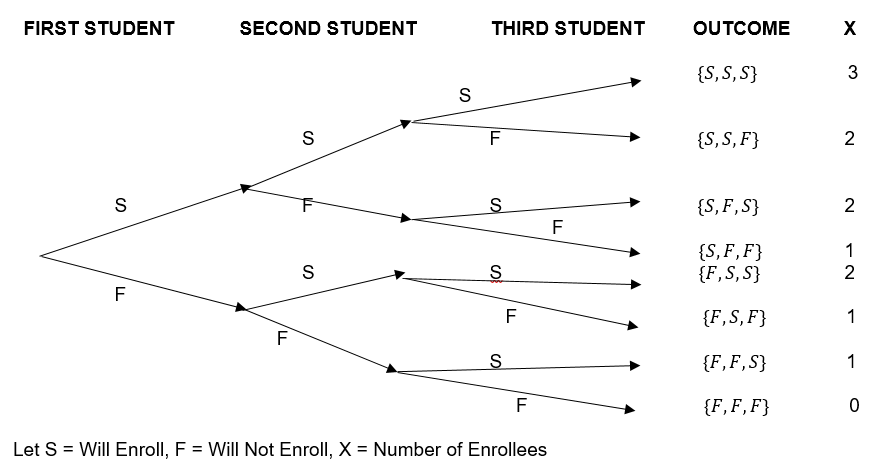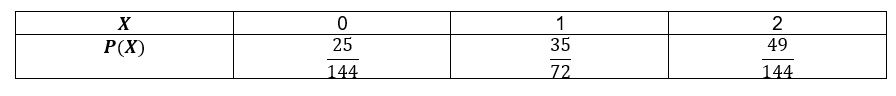# Precalculus - Binomial Distributions

## Introduction

• Binomial Distribution is a type of discrete probability distribution that is related by multiple-step experiments called binomial experiments.
• If a discrete random variable X is used to count the number of successes that occurs in $n$ Bernoulli trials, then this random variable is called a binomial random variable, and the sequence of $n$ Bernoulli trials is said to be a Binomial experiment.
• The binomial experiment involves independent successive trials that generate the probability of success and a probability of failure.
• The probability mass function that generates a probability value to each event in the sample space defined by a binomial random variable, then it forms a Binomial Probability Distribution.
• Binomial experiments should satisfy the following conditions:

1. There are two possible outcomes in each trial only. We consider one outcome as "Success" and the other one as "Failure." The probability of success is denoted by $p$ while the probability of failure is denoted by .

2. The experiment involves successive independent $n$ trials.

3. The probability values do not change from one trial to another trial.

• The probability mass function of a binomial distribution is given by
• In the given formula, $n$ = number of trials, $x$ = number of success elements, $p$ = probability of success, $q$ = probability of failure, $\left(\begin{array}{c}n\\ x\end{array}\right)$= Combination notation similar to $C\left(n,x\right)=\frac{n!}{x!\left(n-x\right)!}$

## Binomial Probability Distributions, Binomial Theorem, and Other Related Concepts

Illustrative Example. Consider the enrollment decisions of the next three students who inquire about MPS Senior High School in Florida. Based on past records, the school registrar estimates the probability that any student will enroll in STEM (Science, Technology, Engineering, and Mathematics) is 0.45. What is the probability that two of the next three students will enroll in STEM at the said school in Florida?

Solution: We need to first check the conditions in order to qualify for a binomial experiment.

1. The test can be described in terms of three consecutive identical trials. That is, one trial per student who will inquire to the school.

2. There are two possible outcomes only - the student will enroll (success) or the student will not enroll (failure).

3. The probability associated with success is 0.45 while the probability of failure is 0.55. This is assumed to be consistent with all possible enrollees.

4. The enrollment decision of each student is independent of the decisions of other students.The number of experimental outcomes in $n$ trials can be computed using the concept of Combinations.

Let's say we want to know the number of experimental outcomes involving two successive trials. This means we get the number of ways of finding the value $X=2$

By computation, we have: $C\left(3,2\right)=\frac{3!}{2\left(3-2\right)!}=3$

This shows that we have: .

To determine the probability of success, we have the following:

$\left\{S,S,F\right\}=\left(p\right)\left(p\right)\left(1-p\right)=\left(0.45\right)\left(0.45\right)\left(0.55\right)=0.111375$

$\left\{S,F,S\right\}=\left(p\right)\left(1-p\right)\left(p\right)=\left(0.45\right)\left(0.55\right)\left(0.45\right)=0.111375$

$\left\{F,S,S\right\}=\left(1-p\right)\left(p\right)\left(p\right)=\left(0.55\right)\left(0.45\right)\left(0.45\right)=0.111375$

By addition rule, we have: .

This implies that the total probability of two of the next three students will enroll in the STEM strand of the said school in Florida is 0.33.

The probability of a particular successive trial outcome with X as the number of successes and $n$ trials is determined by: $P={p}^{x}{\left(1-p\right)}^{n-x}$

The calculation above can be facilitated efficiently by the formula of the Binomial Probability Distribution:

$P\left(X=3\right)=\left(\begin{array}{c}3\\ 2\end{array}\right){\left(0.45\right)}^{2}{\left(0.55\right)}^{3-2}$

Descriptive Statistics Measures

The Mean (Expected Value), Variance, and Standard Deviation of a Binomial Probability Distribution are as follows:

Mean (Expected Value):

Variance:

Standard Deviation: $\sigma =\sqrt{np\left(1-p\right)}$

The Binomial Theorem

The expansion of the binomial ${\left(a+b\right)}^{n}$ for any positive integral values of $n$ is given by ${\left(a+b\right)}^{n}=\sum _{k=0}^{n}\left(\begin{array}{c}n\\ k\end{array}\right){a}^{n-k}{b}^{k}$.

Notice that the binomial theorem formula is closely related to the probability mass function of the binomial distribution, $P\left(X=x\right)=\left(\begin{array}{c}n\\ x\end{array}\right){p}^{x}{q}^{n-x}$

This is based on the fact that two formulas were constructed on the same mathematical procedures. It is simply counting the number of $n$ trials where each trial contains two possible outcomes.

Another way of showing the expansion of the binomial ${\left(a+b\right)}^{n}$ is as follows:

${\left(a+b\right)}^{n}={a}^{n}+n{a}^{n-1}b+\frac{n\left(n-1\right)}{2!}{a}^{n-2}{b}^{2}+\frac{n\left(n-1\right)\left(n-2\right)}{3!}{a}^{n-3}{b}^{3}...+na{b}^{n-1}+{b}^{n}$

To expand a binomial, the use of Pascal's Triangle helps you determine the coefficients in the expansion of the given binomial raised to a positive integral exponent $n$.

Some important properties of the Binomial Expansion.

1. There are $\left(n+1\right)$ terms in the expansion.

2. To find the rth term of the binomial expansion, we use the formula .

3. If $n$ is a positive odd integer, there are two middle terms. If $n$ is a positive even integer, there is only one middle term.

4. To find the sum of the exponents of a binomial expansion, we use the formula:

5. To find the number of terms in a binomial expansion or polynomial expansion, we use the formula:

Finding the Number of Subsets and Proper Subsets

Suppose that a set has $n$ elements, then the number of subsets in the given set is given by ${2}^{n}$ and the number of proper subsets can be determined using the expression ${2}^{n}-1$.

Example: If , then count and write all the possible subsets of $X$.

Number of Subsets subsets

These subsets are

## Solved Examples

Example 1. Suppose that a random variable $X$ follows a binomial distribution. If $p=0.20$ and $n=5$, compute the probabilities $P\left(X=2\right)$ and $P\left(X\ge 4\right)$

Solution: Use the probability mass function $P\left(X=x\right)=\left(\begin{array}{c}n\\ x\end{array}\right){p}^{x}{q}^{n-x}$.

$P\left(X=2\right)=\left(\begin{array}{c}5\\ 2\end{array}\right){\left(0.20\right)}^{2}{\left(0.80\right)}^{5-2}=\frac{128}{625}$

$P\left(X\ge 4\right)=P\left(X=4\right)+P\left(X=5\right)$

$P\left(X=4\right)=\left(\begin{array}{c}5\\ 4\end{array}\right){\left(0.20\right)}^{4}{\left(0.80\right)}^{5-4}=\frac{4}{625}$

$P\left(X=5\right)=\left(\begin{array}{c}5\\ 5\end{array}\right){\left(0.20\right)}^{5}{\left(0.80\right)}^{5-5}=\frac{1}{3125}$

$P\left(X\ge 4\right)=\frac{4}{625}+\frac{1}{3125}=\frac{21}{3125}$

Example 2. An urn contains 7 red balls and 5 blue balls. If two balls are to be drawn successively at random with replacement and X gives the number of red balls, construct a probability distribution for X. What are the mean, variance, and standard deviation of the given distribution?

Solution: Using the Binomial Probability Distribution

Let X be the random variable that gives the number of red balls. The probability mass function is $P\left(X=x\right)=\left(\begin{array}{c}n\\ x\end{array}\right){p}^{x}{\left(1-p\right)}^{n-x}$.

In this example, we have

If $X=0$, then $P\left(X=0\right)=\left(\begin{array}{c}2\\ 0\end{array}\right){\left(\frac{7}{12}\right)}^{0}{\left(\frac{5}{12}\right)}^{2-0}=\frac{25}{144}$

If $X=1$, then $P\left(X=1\right)=\left(\begin{array}{c}2\\ 1\end{array}\right){\left(\frac{7}{12}\right)}^{1}{\left(\frac{5}{12}\right)}^{2-1}=\frac{35}{72}$

If $X=2$,, then $P\left(X=2\right)=\left(\begin{array}{c}2\\ 2\end{array}\right){\left(\frac{7}{12}\right)}^{2}{\left(\frac{5}{12}\right)}^{2-2}=\frac{49}{144}$

Thus, we have the probability distribution for X below:To compute the expected value (mean), we use

$E\left(x\right)=\left(2\right)\left(\frac{7}{12}\right)=\frac{7}{6}$

For the variance, we have:

${\sigma }^{2}=npq$

${\sigma }^{2}=\left(2\right)\left(\frac{7}{12}\right)\left(\frac{5}{12}\right)=\frac{35}{72}$

The standard deviation is simply the square root of the variance: $\sigma =\sqrt{\frac{35}{72}}\approx 0.6972$

Example 3. Expand the binomial ${\left(2x-5\right)}^{5}$ using the Binomial Theorem.

${\left(2x-5\right)}^{5}={\left(2x\right)}^{5}-5{\left(2x\right)}^{4}\left(5\right)+\frac{5×4}{2!}{\left(2x\right)}^{3}{\left(5\right)}^{2}-\frac{5×4×3}{3!}{\left(2x\right)}^{2}{\left(5\right)}^{3}+\frac{5×4×3×2}{4!}{\left(2x\right)}^{1}{\left(5\right)}^{4}-\frac{5×4×3×2×1}{5!}{\left(2x\right)}^{0}{\left(5\right)}^{5}$

${\left(2x-5\right)}^{5}=32{x}^{5}-400{x}^{4}+2000{x}^{3}-5000{x}^{2}+6250x-3125$

Using the concept of Combinations, we can generate the same expanded form:

## Cheat Sheet

• Problems involving independent identical $n$ "with replacement" involve Binomial Distribution.
• To compute the mean (expected value) of a binomial probability distribution, the alternative formula is $E\left(x\right)=\sum _{}x·P\left(x\right)$. This is applicable when the distribution is already given.
• In the binomial expansion ${\left(a+b\right)}^{n}$, the exponent of $a$ decreases by 1 as we proceed from term to term. On the other hand, the exponent of $b$ increases as we proceed to successive terms.
• When the binomial ${\left(x-y\right)}^{n}$ is expanded, the sign of each succeeding term alternates.

## Blunder Areas

• Problems involving $n$ trials yet dependent events involve Hypergeometric Probability Distribution and not Binomial Distribution.
• The term "without replacement" used in probability problems does not involve Binomial Distribution.
• Binomial Probability Distribution and Hypergeometric Probability Distribution have the same notion of having the probability of success and the probability of failure.
• Binomial Probability Distribution is related to the probability of discrete random variables. Thus, this distribution does not hold for continuous random variables such as height, weight, test scores, and the like.
• Normal Distribution and Binomial Distribution are related in the notion that .